Function Repository Resource:

Get a canonical form for an overlap tiling mask

Contributed by: Wolfram Research

## Details

In this code, the mask for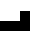is {{0,0,1},{1,1,1}}. Below, the shapes are given instead of the arrays as a visual aid for the reader:
This function is very specific to the overlap tilings seen in the resource function FindMinimalTilings.
A tile mask is a subset of positions within a rectangular array, such as the a values in {{a,a,a},{_,a,_}}. This particular mask is also known as the Tetris T shape,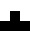.
The results of FindMinimalTilings on maskhas identical results to rotations and reflections of.
The results of running FindMinimalTilings on maskhas identical results to mask(skew equivalency).
Similarly,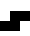and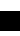are equivalent masks for overlap tiling systems.
Evaluating ResourceFunction["CanonicalTilingMask"] on any rotation or reflection of masks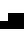,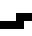or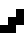returns.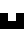,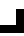and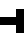are canonical.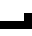is the canonical form of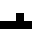.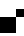is the canonical form of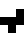.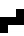is the canonical form of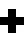.
Currently, many hours of computer time are needed to run FindMinimalTilings on masks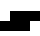,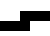and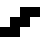. These are all equivalent to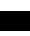. The point of this function is to find these equivalencies before an expensive run.
For overlap tiling systems, these results only meaningfully apply to 2D shapes. The domino array {{1,1}} can be considered a 1D mask.

## Examples

### Basic Examples (2)

The canonical tiling mask for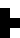is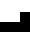:

 In:=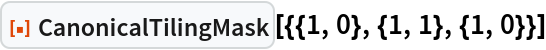Out=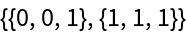In:=Out=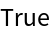### Scope (1)

Find canonical masks for the pentomino shapes:

 In:=Out=### Neat Examples (3)

Find canonical masks (bottom row) for the hexomino shapes (top row):

 In:=Out=Find a canonical mask for a random heptomino:

 In:=Out=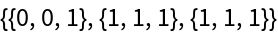For masks using five bits and having a maximum dimension of 4, there are 192 canonical cases, shown below:

 In:=Out=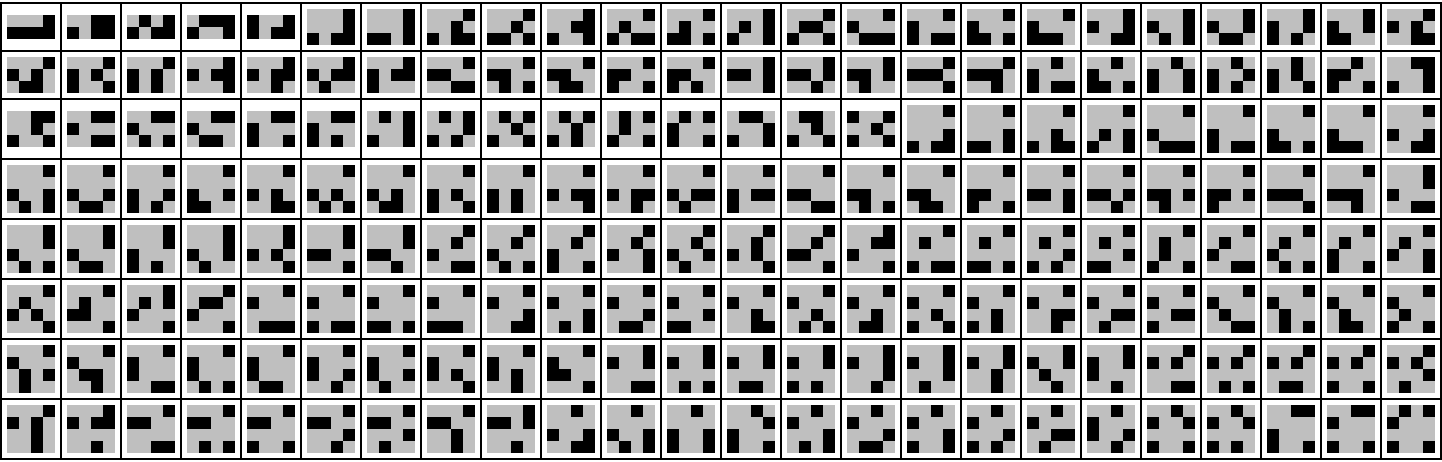An exercise to test them all is left as an exercise for the reader. Meanwhile, here we test three of these at random:

 In:=Out=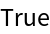## Version History

• 1.0.0 – 29 July 2022